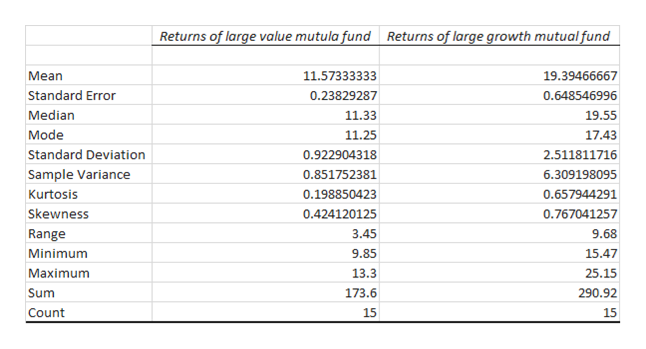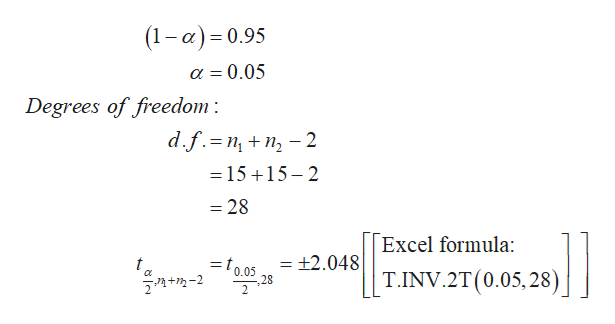# See the graph of data below to answer the following questionObtain a 95% confidence interval for the difference between the mean rates of return.  Interpret the interval.

Question
9 views

See the graph of data below to answer the following question

Obtain a 95% confidence interval for the difference between the mean rates of return.  Interpret the interval.

check_circle

star
star
star
star
star
1 Rating
Step 1

Procedure to find sample mean, standard deviation and sample size using Excel:

• In Excel sheet, enter Returns of large value mutual fund and Returns of large growth mutual fund in different columns.
• In Data, select Data Analysis and Choose Descriptive Statistics.
• In Input Range, select Returns of large value mutual fund and Returns of large growth mutual fund and click Labels in First Row.
• Select Summary Statistics.
• Click OK.

Output using Excel is shown below:help_outlineImage TranscriptioncloseReturns of large value mutula fund Returns of large growth mutual fund 19.39466667 Mean 11.57333333 Standard Error 0.23829287 0.648546996 Median 11.33 19.55 Mode 11.25 17.43 Standard Deviation 2.511811716 0.922904318 Sample Variance 0.851752381 6.309198095 0.198850423 Kurtosis 0.657944291 Skewness 0.424120125 0.767041257 Range 3.45 9.68 9.85 Minimum 15.47 Maximum 13.3 25.15 Sum 173.6 290.92 Count 15 15 fullscreen
Step 2

Here, n1 = 15, n2 = 15, s12 is 0.8518 and s22  is 6.3092.

Find the pooled sample standard deviation:

Step 3

Obtain the critical value:

The required confidence level is 100*(1–α)% = 95%.

That is, the level o...help_outlineImage Transcriptionclose|(1-a) 0.95 0.05 Degrees of freedom d.f. nn2 =15 15-2 = 28 Excel formula: = ±2.048 t zl0.05 28 2. T.INV.2T(0.05,28) +-2 fullscreen

### Want to see the full answer?

See Solution

#### Want to see this answer and more?

Solutions are written by subject experts who are available 24/7. Questions are typically answered within 1 hour.*

See Solution
*Response times may vary by subject and question.
Tagged in

### Statistics